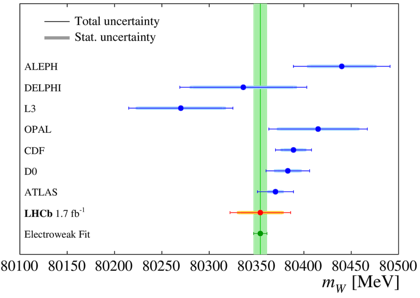# The first LHCb measurement of the W boson mass##### [ mW=80354±23stat±10expsys±17theory±9PDF MeV]

Last week the LHCb Collaboration submitted for publication a paper that reports the first LHCb measurement of the W boson mass with an uncertainty of 32 MeV using the 2016 data set. A more precise measurement with a total uncertainty of ≲ 20 MeV looks achievable with already existing LHCb data.

One of the common decays of a W boson is into a muon and a neutrino. The muon μ transverse momentum distribution, or better 1/pT, was selected as the best way to measure the W boson mass, mW, in this analysis. The shape of this distribution is related the value of mW but it is also strongly influenced by the W boson transverse momentum distribution, pTW, the modelling of which is a potential source of a limiting systematic uncertainty. At the LHC W bosons are produced in the collisions of partons (quarks, gluons) carrying a fraction x of proton momentum encoded in the parton distribution functions, PDFs.

The left image above shows the q/pT distribution, where q is the muon charge. In this way muons from the decay of positive W+ and negative W bosons are seen in different part of the image. LHCb physicists decided to use measurements of the transverse momentum distribution for Z boson production, pTZ, in order to validate (calibrate) the predictions for the pTW distribution. The right image above shows the distribution of the φ* variable, defined in the paper, and strongly related to the pTZ distribution. The W boson mass is measured in the simultaneous analysis (fits) of the q/pT distribution of muons from the W boson decays and the φ* distribution from the Z boson decays. Note that the Z boson mass is known with the very high precision of 2.1 MeV. This method has strongly reduced sensitivity to the uncertainties in modelling the W boson transverse momentum distribution. The W mass was measured with the three different parton distribution functions, PDFs. Treating them equally leads to the following arithmetic average: mW=80354±23stat±10expsys±17theory±9PDF MeV.

The charged W bosons were discovered, together with their neutral partner Z boson, by the UA1 and UA2 experiments at CERN in 1983. Their experimental discovery was crucial in establishing what is now called the Standard Model (SM) of particle physics. In the SM the values of different parameter are related to each other. Precisely measured values of some parameters fix (predict) values of other not well measured or unknown parameters. In this way the value of the Higgs boson mass was predicted before the Higgs boson was discovered by the ATLAS and CMS experiments at CERN in 2012. The value of the W mass was one of the most important parameters used in this prediction. The W boson mass was measured to a precision of 33 MeV at the Large Electron-Positron (LEP) collider at CERN from a combination of results obtained by the ALEPH, DELPHI, L3 and OPAL experiments and to a precision of 16 MeV in an average of measurements by the CDF and D0 experiments at the Fermilab Tevatron collider. The first measurement at the LHC was performed by the ATLAS collaboration and has an uncertainty of 19 MeV. The current SM prediction of the W boson mass is mW=80354±7 MeV, based on the combination of a large number of SM measurements except that of mW, while the average of direct measurements is mW=80379±12 MeV. Since all the SM particles are now discovered, the differences between the SM predictions and direct measurements are used to test the completeness of the SM and could indicate the presence of the physics beyond the SM if significant discrepancies are identified. The mW comparison is currently primarily limited by the precision of the direct measurements of mW. The image above, obtained by the Gfitter group, shows the compatibility of the SM predictions in blue with the direct measurements of the W boson and top quark masses in yellow.

The image to the left shows the comparison of the LHCb result, obtained using the 2016 data set, with those of LEP, Tevatron and ATLAS experiments as well as with the SM prediction marked as “Electroweak Fit”. A future analysis will use the full available LHCb data set, with which a W mass measurement could be made with an uncertainty of 20 MeV or better. Further reduction could be obtained in collaboration with theoretical physicists by improving the modeling of the W boson transverse momentum distribution. The combination of LHCb results with those from ATLAS and CMS experiments will reduce the sensitivity to the parton distribution functions, PDFs. In fact, in the unique forward geometry of LHCb the W bosons are produced in collisions of high- with low-x partons, while collisions of mid-x partons mainly produce the W bosons observed at ATLAS and CMS.

Read more in the LHCb paper. These results have been already presented in CERN seminar and EPS conference presentations  and .